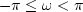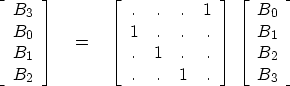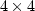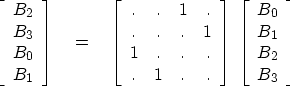Next: TWO-DIMENSIONAL FT Up: SETTING UP THE FAST Previous: SETTING UP THE FAST

## Shifted spectra

Customarily, FT programs store frequencies in the interval.In some applications the intervalis preferable, and here we will see how this shift in one domain can be expressed as a product in the other domain. First we examine shifting by matrix multiplication. A single unit shift, wrapping the end value around to the beginning, is(15)

You might recognize that equation (15) convolves a wavelet with a delayed impulse, where the bottom of the matrix is wrapped back in to the top to keep the output the same length as the input. For thismatrix, shifting one more point does the job of switching the high and low frequencies:(16)
We are motivated to seek an algebraic identity for thematrix which represents the fact that convolution in the time domain is multiplication in the frequency domain. To this end we will look at the converse theorem, that multiplication in the time domain does shifting in the frequency domain. On the left of equation (17) is the operation that first transforms from time to frequency and then swaps high and low frequencies. On the right is the operation that weights in the time domain, and then Fourier transforms. To verify the equation, multiply the matrices and simplify with W4=1 to throw out all powers greater than 3.(17)

For an FT matrix of arbitrary size N, the desired shift is N/2, so values at alternate points in the time axis are multiplied by -1. A subroutine for that purpose is fth().

# FT a vector in a matrix, with first omega = - pi
#
subroutine  fth( adj,sign, m1, n12, cx)
real sign
complex cx(m1,n12)
temporary complex temp(n12)
do i= 1, n12
temp(i) = cx(1,i)
if( adj == 0)   { do i= 2, n12, 2
temp(i) =  -temp(i)
call ftu(  sign, n12, temp)
}
else            { call ftu( -sign, n12, temp)
do i= 2, n12, 2
temp(i) =  -temp(i)
}
do i= 1, n12
cx(1,i) = temp(i)
return; end


To Fourier transform a 1024-point complex vector cx(1024) and then inverse transform it, you would

call fth( 0, 1., 1, 1024, 1, cx)
call fth( 1, 1., 1, 1024, 1, cx)


You might wonder about the apparent redundancy of using both the argument conj and the argument sign. Having two arguments instead of one allows us to define the forward transform for a time axis with the opposite sign as the forward transform for a space axis.

The subroutine fth() is somewhat cluttered by the inclusion of a frequently needed practical feature--namely, the facility to extract vectors from a matrix, transform the vectors, and then restore them into the matrix.Next: TWO-DIMENSIONAL FT Up: SETTING UP THE FAST Previous: SETTING UP THE FAST
Stanford Exploration Project
10/21/1998